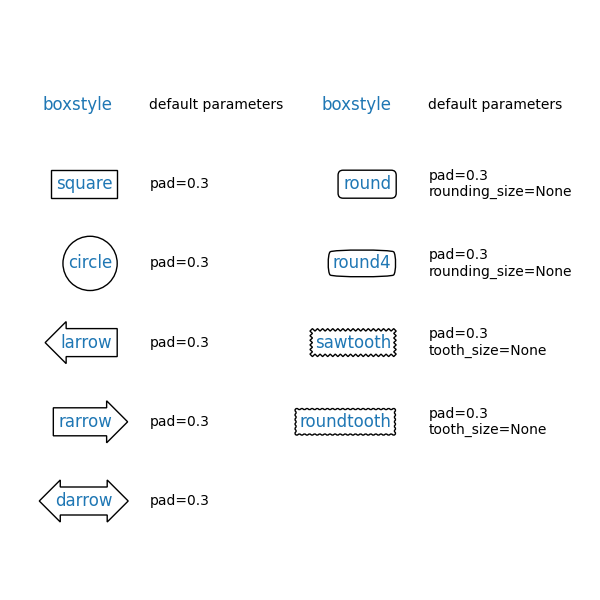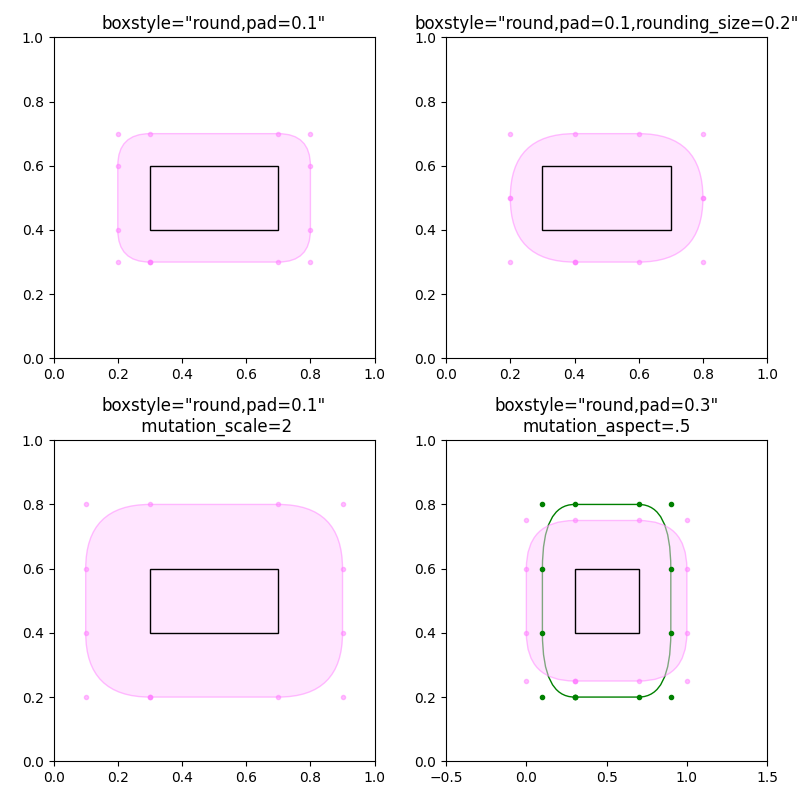# Drawing fancy boxes¶

The following examples show how to plot boxes with different visual properties.

import inspect

import matplotlib.pyplot as plt
import matplotlib.transforms as mtransforms
import matplotlib.patches as mpatch
from matplotlib.patches import FancyBboxPatch


First we'll show some sample boxes with fancybox.

styles = mpatch.BoxStyle.get_styles()
ncol = 2
nrow = (len(styles) + 1) // ncol
axs = (plt.figure(figsize=(3 * ncol, 1 + nrow))
for ax in axs.flat:
ax.set_axis_off()
for ax in axs[0, :]:
ax.text(.2, .5, "boxstyle",
transform=ax.transAxes, size="large", color="tab:blue",
horizontalalignment="right", verticalalignment="center")
ax.text(.4, .5, "default parameters",
transform=ax.transAxes,
horizontalalignment="left", verticalalignment="center")
for ax, (stylename, stylecls) in zip(axs[1:, :].T.flat, styles.items()):
ax.text(.2, .5, stylename, bbox=dict(boxstyle=stylename, fc="w", ec="k"),
transform=ax.transAxes, size="large", color="tab:blue",
horizontalalignment="right", verticalalignment="center")
ax.text(.4, .5, str(inspect.signature(stylecls))[1:-1].replace(", ", "\n"),
transform=ax.transAxes,
horizontalalignment="left", verticalalignment="center")Next we'll show off multiple fancy boxes at once.

def add_fancy_patch_around(ax, bb, **kwargs):
fancy = FancyBboxPatch((bb.xmin, bb.ymin), bb.width, bb.height,
fc=(1, 0.8, 1, 0.5), ec=(1, 0.5, 1, 0.5),
**kwargs)
return fancy

def draw_control_points_for_patches(ax):
for patch in ax.patches:
patch.axes.plot(*patch.get_path().vertices.T, ".",
c=patch.get_edgecolor())

fig, axs = plt.subplots(2, 2, figsize=(8, 8))

# Bbox object around which the fancy box will be drawn.
bb = mtransforms.Bbox([[0.3, 0.4], [0.7, 0.6]])

ax = axs[0, 0]
# a fancy box with round corners. pad=0.1
ax.set(xlim=(0, 1), ylim=(0, 1), aspect=1,

ax = axs[0, 1]
# bbox=round has two optional arguments: pad and rounding_size.
# They can be set during the initialization.
# The boxstyle and its argument can be later modified with set_boxstyle().
# Note that the old attributes are simply forgotten even if the boxstyle name
# is same.
ax.set(xlim=(0, 1), ylim=(0, 1), aspect=1,

ax = axs[1, 0]
# mutation_scale determines the overall scale of the mutation, i.e. both pad
# and rounding_size is scaled according to this value.
ax.set(xlim=(0, 1), ylim=(0, 1), aspect=1,

ax = axs[1, 1]
# When the aspect ratio of the axes is not 1, the fancy box may not be what you
# expected (green).
fancy.set(facecolor="none", edgecolor="green")
# You can compensate this by setting the mutation_aspect (pink).
ax.set(xlim=(-.5, 1.5), ylim=(0, 1), aspect=2,

for ax in axs.flat:
draw_control_points_for_patches(ax)
# Draw the original bbox (using boxstyle=square with pad=0).
fancy.set(edgecolor="black", facecolor="none", zorder=10)

fig.tight_layout()

plt.show()References

The use of the following functions, methods, classes and modules is shown in this example:

Total running time of the script: ( 0 minutes 1.601 seconds)

Keywords: matplotlib code example, codex, python plot, pyplot Gallery generated by Sphinx-Gallery